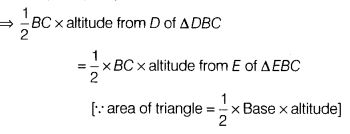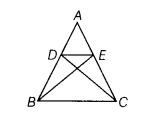# Suppose D and E are the points on sides AB and AC respectively of ∆ ABC

Suppose D and E are the points on sides AB and AC respectively of ∆ ABC such that
ar (DBC) = ar (EBC). Prove that DE || BC.

Given, a ∆ ABC and D and E are the points on sides AB and AC respectively, also
ar (DBC) = ar (EBC)So, altitude from D of ∆ DBC=altitude from E of ∆EBCHence, ∆ DBC and ∆ EBC are between the same parallels, i.e. DE || BC.